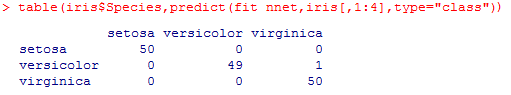R语言高级算法之人工神经网络（Artificial Neural Network）

1.人工神经网络原理分析:

2.在R语言中的应用

nnet(formula,data,weights,size,...,subset,na.action,contrasts=NULL)

3.以iris数据集为例进行判别分析
1)应用模型并观察输出结果

fit_nnet=nnet(Species~.,data=iris,size=4,decay=5e-4,maxit=200)fit_nnet[1:length(fit_nnet)]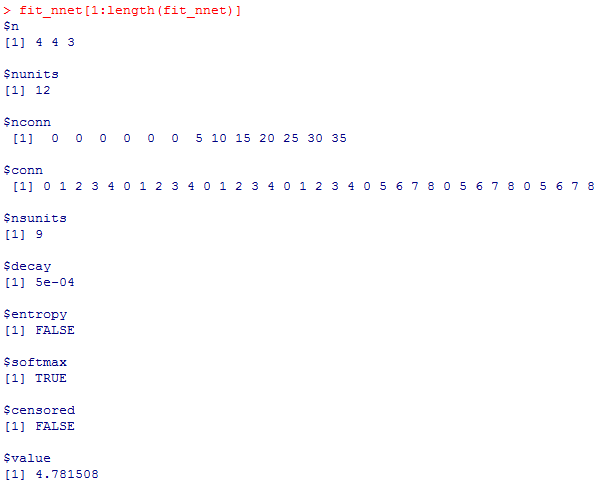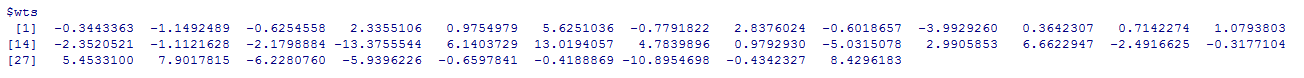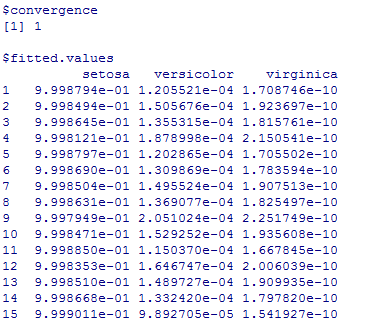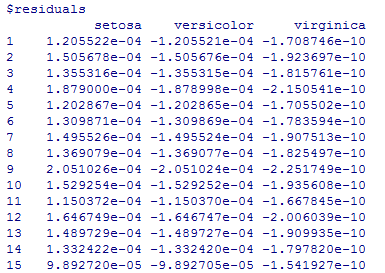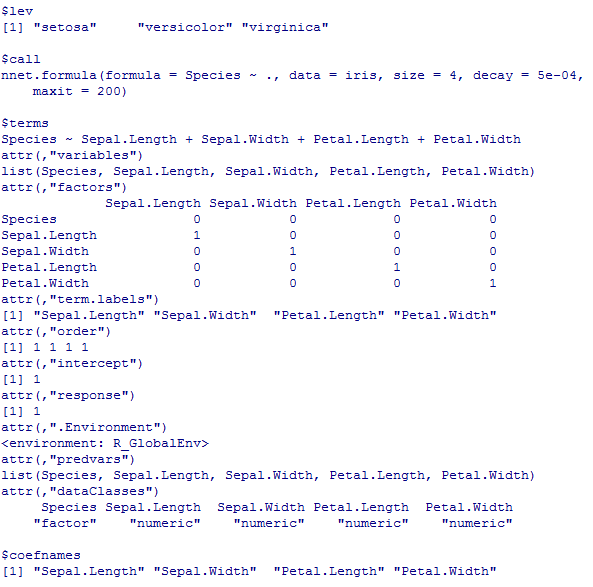3)检测模型准确度

predict(fit_nnet,iris[,1:4],type="class")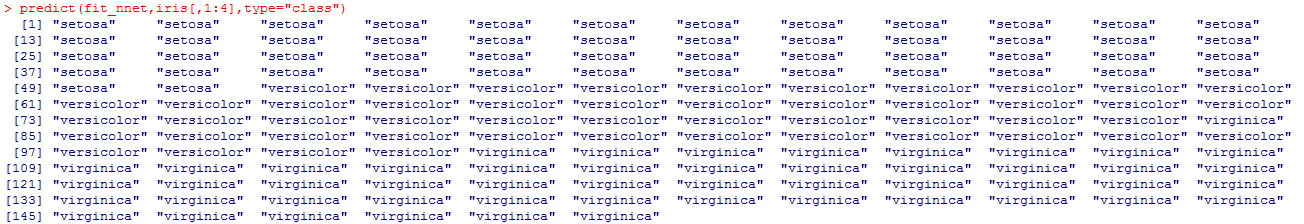table(iris\$Species,predict(fit_nnet,iris[,1:4],type="class"))